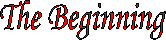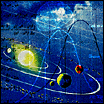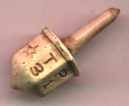Since participation in games and gambling is as old as mankind, it seems as if the idea of probability should be almost as old. But, the realization that one could predict an outcome to a certain degree of accuracy was unconceivable until the sixteenth and seventeenth centuries. This idea that one could determine the chance of a future event was prompted from the necessity to achieve a predictable balance between risks taken and the potential for gain. In order to make a profit, underwriters were in need of dependable guidelines by which a profit could be expected, while the gambler was interested in predicting the possibility of gain.The first recorded evidence of probability theory can be found as early as 1550 in the work of Cardan. In 1550 Cardan wrote a manuscript in which he addressed the probability of certain outcomes in rolls of dice, the problem of points, and presented a crude definition of probability. Had this manuscript not been lost, Cardan would have certainly been accredited with the onset of probability theory. However, the manuscript was not discovered until 1576 and printed in 1663, leaving the door open for independent discovery.      The onset of probability as a useful science is primarily attributed to Blaise Pascal (1623-1662) and Pierre de Fermat (1601-1665). While contemplating a gambling problem posed by Chevalier de Mere in 1654, Blaise Pascal and Pierre de Fermat laid the fundamental groundwork of probability theory, and are thereby accredited the fathers of probability. The question posed was pertaining to the number of turns required to ensure obtaining a six in the roll of two dice. The correspondence between Pascal and Fermat concerning this and the problem of points led to the beginning of the new concepts of probability and expection.In the seventeenth century, a shopkeeper, John Graunt (1620-1674), set out to predict mortality rates by categorizing births and deaths. In the London Life Table, Graunt made a noteworthy attempt to predict the number of survivors out of one hundred through increments of ten years. This work along with his earlier paper Natural and Political Observations Made upon the Bills of Mortality, the first known paper to use data in order to draw statistical inferences, gained him access into the Royal Society of London.       Graunt’s observations and predictions elicited interest in probability from others, such as two brothers, Ludwig and Christiaan Huygens. Beginning with the interest initially sparked by Graunt’s work and later by the work of Pascal and Fermat, Christiaan Huygens, a Dutch physicist, became the first to publish a text on probability theory entitled De Ratiociniis in Ludo Aleae (On Reasoning in Games and chance), in 1657. In this text, Huygens presented the idea of mathematical expectation. This text was unrivaled until James Bernoulli (1654-1705) wrote Ars Conjectandi, which was published eight years after his death.       In Ars Conjectandi, Bernoulli expounded on and provided alternative proofs to Huygens’ De Ratiociniis in Ludo Aleae, presented combinations and permutations which encompass most of the results still used today, included a series of problems on games of chance with explanations, and finally, and most importantly, he revealed the famous bernoulli theorem, later called the law of large numbers.       Probability theory continued to grow with Abraham DeMoivre’s Doctrine of Chances: or, a Method of Calculating the Probability of Events in Play, published in 1718, and Pierre Simon Laplace’s (1749-1827) Theorie Analytique des Probabilites, published in 1812. The Theorie Analytique des Probabilites outlined the evolution of probability theory, providing extensive explanations of the results obtained. In this book Laplace presented the definition of probability, which we still use today, and the fundamental theorems of addition and multiplication of probabilities along with several problems applying the Bernoulli process.       The first major accomplishment in the development of probability theory was the realization that one could actually predict to a certain degree of accuracy events which were yet to come. The second accomplishment, which was primarily addressed in the 1800's, was the idea that probability and statistics could converge to form a well defined, firmly grounded science, which seemingly has limitless applications and possibilities. It was the initial work of Pascal, Fermat, Graunt, Bernoulli, DeMoivre, and Laplace that set probability theory, and then statistics, on its way to becoming the valuable inferential science that it is today.